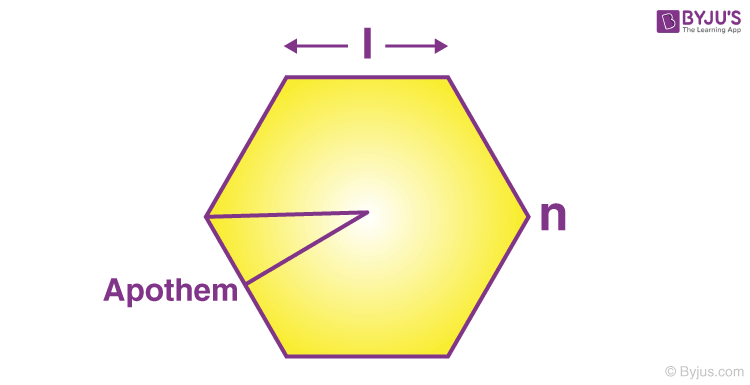# Area of Regular Polygon Formula

A polygon having equal sides, i.e. equilateral and equal angles i.e. equiangular is known as a regular polygon. An apothem is also used sometimes to find the area of a regular polygon. Apothem is a segment that joins the polygon’s center to the midpoint of any side and it is perpendicular to that side. All vertices of a regular polygon lie on a common circle (the circumscribed circle), i.e., they are concyclic points. That is, a regular polygon is a cyclic polygon. The property of equal-length sides implies that every regular polygon also has an inscribed circle or incircle that is tangent to every side at the midpoint. Thus, a regular polygon is a tangential polygon.

A regular n-sided polygon can be constructed with compass and straightedge if and only if the odd prime factors of n are distinct Fermat primes. See constructible polygon.The formula for the area of a regular polygon is given as,

#### A =$$\begin{array}{l}\frac{l^{2}n}{4tan(\frac{\pi }{n})}\end{array}$$

Where,
l is the side length
n is the number of sides

### Solved Example

Question 1: Calculate the area of 5 sided polygon with a side length 4 cm.
Solution:

The given parameters are,
l = 4 cm and n = 5
The formula for finding the area is,
A =
$$\begin{array}{l}\frac{l^{2}n}{4tan(\frac{\pi }{n})}\end{array}$$
A =
$$\begin{array}{l}\frac{4^{2}\times5}{4tan(\frac{180}{5})}\end{array}$$
A =
$$\begin{array}{l}\frac{80}{4\times0.7265}\end{array}$$
A = 27.53 cm2